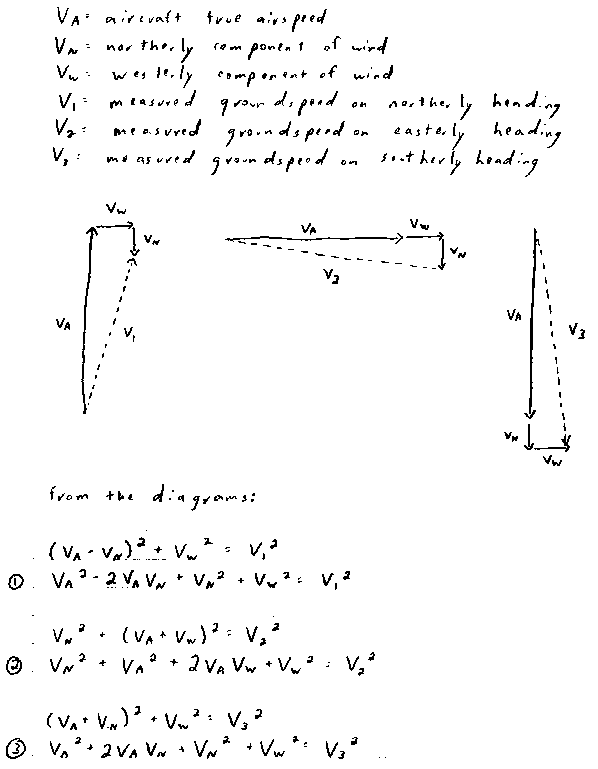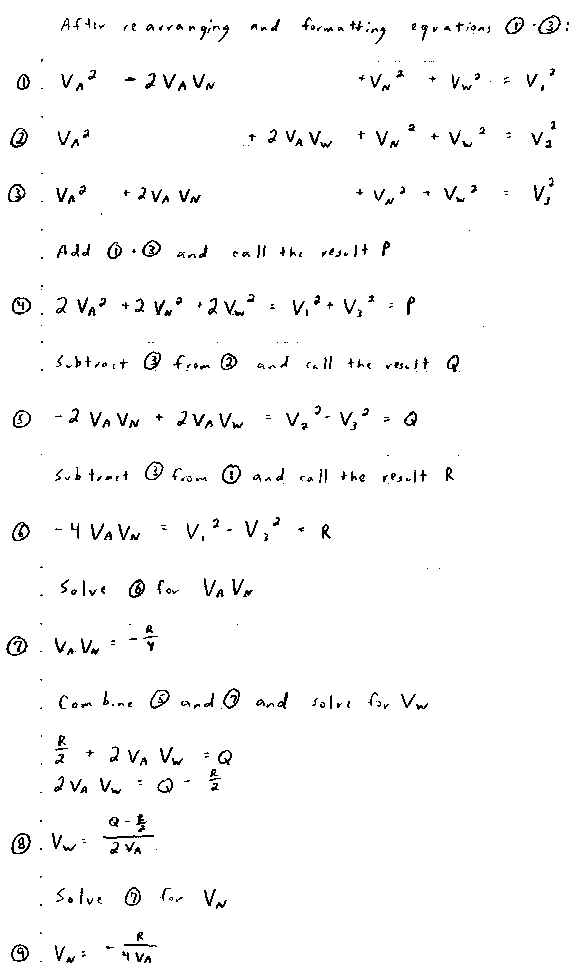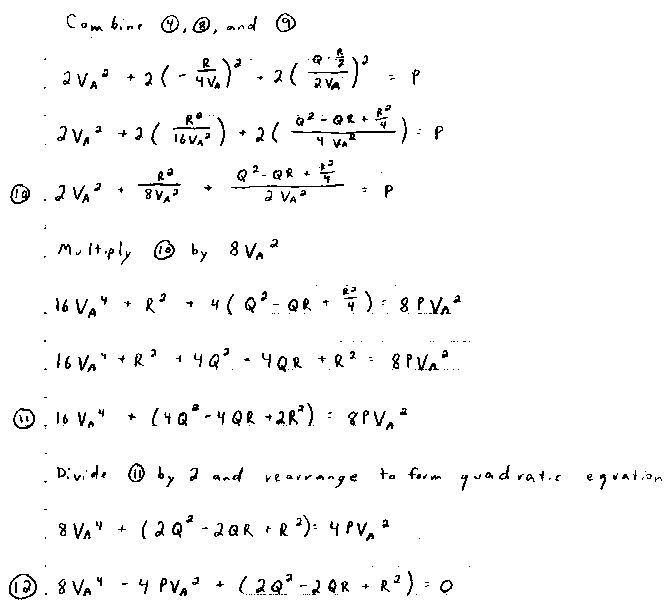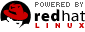# True Airspeed Equations

The following equations show the derivation of a quadratic equation to determine true airspeed given three GPS groundspeed readouts on headings of north, east, and south. Most of these equations are used for the derivation itself and are NOT used when actually determining a particular true airspeed. Here are the steps to determine a particular true airspeed.
1. Obtain the GPS groundspeeds on headings of north, east, and south and call them V1, V2, and V3, respectively.
2. Square the groundspeeds to obtain V12, V22, and V32.
3. Add V12 to V32 and call the result P.
4. Subtract V32 from V22 and call the result Q.
5. Subtract V32 from V12 and call the result R.
6. Enter the values for P, Q, and R into the final equation, #12.
7. Solve the quadratic equation #12 for VA2 using the quadratic formula. Take the square root of the result to determine VA, the aircraft true airspeed.For comments or questions about this web site or applets, contact the webmaster. For the address of the webmaster, look at the first few comments of a Java file. To obtain all Java files, download the True Airspeed Calculator ZIP file. The True Airspeed Calculator ZIP file is available on the Distribution Policy page. Copyright 2001 REA Computing, Inc.